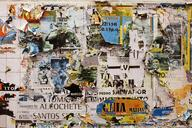## ML From Scratch, Part 6: Principal Component AnalysisIn the previous article in this series we distinguished between two kinds of unsupervised learning (cluster analysis and dimensionality reduction) and discussed the former in some detail. In this installment we turn our attention to the later. In dimensionality reduction we seek a function $$f : \mathbb{R}^n \mapsto \mathbb{R}^m$$ where $$n$$ is the dimension of the original data $$\mathbf{X}$$ and $$m$$ is less than or equal to $$n$$. That is, we want to map some high dimensional space into some lower dimensional space.
Read more...

## ML From Scratch, Part 5: Gaussian Mixture ModelsConsider the following motivating dataset: Unlabled Data It is apparent that these data have some kind of structure; which is to say, they certainly are not drawn from a uniform or other simple distribution. In particular, there is at least one cluster of data in the lower right which is clearly separate from the rest. The question is: is it possible for a machine learning algorithm to automatically discover and model these kinds of structures without human assistance?
Read more...

## Adaptive Basis FunctionsToday, let me be vague. No statistics, no algorithms, no proofs. Instead, we’re going to go through a series of examples and eyeball a suggestive series of charts, which will imply a certain conclusion, without actually proving anything; but which will, I hope, provide useful intuition. The premise is this: For any given problem, there exists learned featured representations which are better than any fixed/human-engineered set of features, even once the cost of the added parameters necessary to also learn the new features into account.
Read more...

## ML From Scratch, Part 4: Decision TreesSo far in this series we’ve followed one particular thread: linear regression -> logistic regression -> neural network. This is a very natural progression of ideas, but it really represents only one possible approach. Today we’ll switch gears and look at a model with completely different pedigree: the decision tree, sometimes also referred to as Classification and Regression Trees, or simply CART models. In contrast to the earlier progression, decision trees are designed from the start to represent non-linear features and interactions.
Read more...

## ML From Scratch, Part 3: BackpropagationIn today’s installment of Machine Learning From Scratch we’ll build on the logistic regression from last time to create a classifier which is able to automatically represent non-linear relationships and interactions between features: the neural network. In particular I want to focus on one central algorithm which allows us to apply gradient descent to deep neural networks: the backpropagation algorithm. The history of this algorithm appears to be somewhat complex (as you can hear from Yann LeCun himself in this 2018 interview) but luckily for us the algorithm in its modern form is not difficult - although it does require a solid handle on linear algebra and calculus.
Read more...

## ML From Scratch, Part 2: Logistic RegressionIn this second installment of the machine learning from scratch we switch the point of view from regression to classification: instead of estimating a number, we will be trying to guess which of 2 possible classes a given input belongs to. A modern example is looking at a photo and deciding if its a cat or a dog. In practice, its extremely common to need to decide between $$k$$ classes where $$k > 2$$ but in this article we’ll limit ourselves to just two classes - the so-called binary classification problem - because generalizations to many classes are usually both tedious and straight-forward.
Read more...

## ML From Scratch, Part 1: Linear RegressionTo kick off this series, will start with something simple yet foundational: linear regression via ordinary least squares. While not exciting, linear regression finds widespread use both as a standalone learning algorithm and as a building block in more advanced learning algorithms. The output layer of a deep neural network trained for regression with MSE loss, simple AR time series models, and the “local regression” part of LOWESS smoothing are all examples of linear regression being used as an ingredient in a more sophisticated model.
Read more...

## ML From Scratch, Part 0: IntroductionMotivation “As an apprentice, every new magician must prove to his own satisfaction, at least once, that there is truly great power in magic.” - The Flying Sorcerers, by David Gerrold and Larry Niven How do you know if you really understand something? You could just rely on the subjective experience of feeling like you understand. This sounds plausible - surely you of all people should know, right? But this runs head-first into in the Dunning-Kruger effect.
Read more...

## Visualizing Multiclass Classification ResultsIntroduction Visualizing the results of a binary classifier is already a challenge, but having more than two classes aggravates the matter considerably. Let’s say we have $k$ classes. Then for each observation, there is one correct prediction and $k-1$ possible incorrect prediction. Instead of a $2 \times 2$ confusion matrix, we have a $k^2$ possibilities. Instead of having two kinds of error, false positives and false negatives, we have $k(k-1)$ kinds of errors.
Read more...